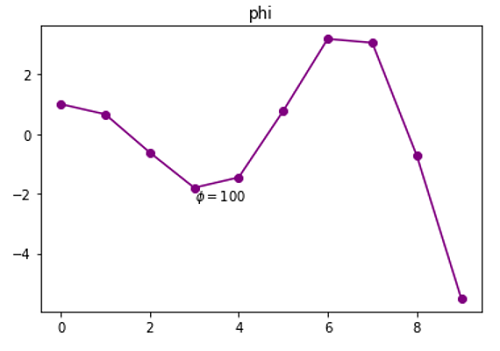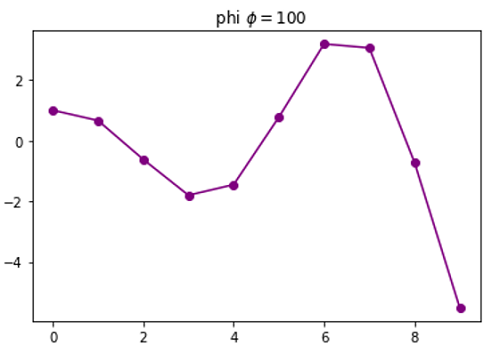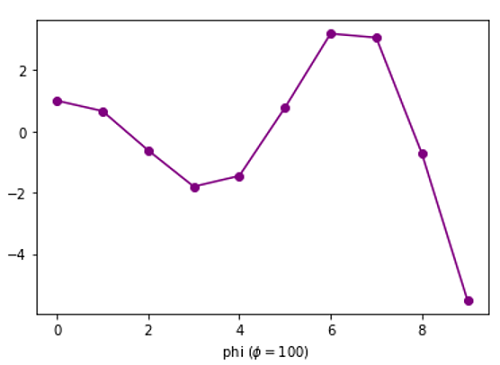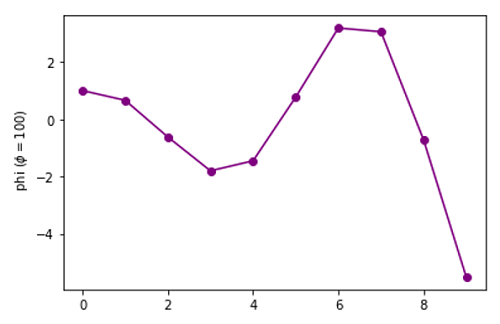# Python | Adding Phi to Plot Label

Here, we are going to learn about the adding phi to plot label and its Python implementation.
Submitted by Anuj Singh, on August 31, 2020

Phi is a very oftenly used greek mathematical letter. They are mostly used in physics and other mathematical expressions, especially, when there is topic related change (for example charge and electrodynamics) and therefore, they have registered an importance in languages.

Therefore, matplotlib has defined a command for usage.

plt.text(3, 0.4, r'$\nabla=100$')plt.title('nabla 'r'$\nabla=100$')
#Adding ɸ in title of the figureplt.xlabel('nabla ('r'$\nabla=100)$')
#Adding ɸ in x axis label of the figureplt.ylabel('nabla ('r'$\nabla=100)$')
#Adding ɸ in y axis label of the figure## Python code for adding phi to plot label

import numpy as np
import matplotlib.pyplot as plt

x = np.arange(10)
y = np.cos(x)*np.exp(x/5)

#  phi
#In text
plt.figure()
plt.plot(x,y, '-o', color=  'purple')
plt.title('phi')
plt.text(3, -2.2, r'$\phi=100$')
plt.show()

#In title
plt.figure()
plt.plot(x,y, '-o', color=  'purple')
plt.title('  phi 'r'$\phi=100$')
plt.show()

#In x-axis label
plt.figure()
plt.plot(x,y, '-o', color=  'purple')
plt.xlabel('  phi ('r'$\phi=100)$')
plt.show()

#In y-axis label
plt.figure()
plt.plot(x,y, '-o', color=  'purple')
plt.ylabel('  phi ('r'$\phi=100)$')
plt.show()


Output:

Output is as Figure


Languages: » C » C++ » C++ STL » Java » Data Structure » C#.Net » Android » Kotlin » SQL
Web Technologies: » PHP » Python » JavaScript » CSS » Ajax » Node.js » Web programming/HTML
Solved programs: » C » C++ » DS » Java » C#
Aptitude que. & ans.: » C » C++ » Java » DBMS
Interview que. & ans.: » C » Embedded C » Java » SEO » HR
CS Subjects: » CS Basics » O.S. » Networks » DBMS » Embedded Systems » Cloud Computing
» Machine learning » CS Organizations » Linux » DOS
More: » Articles » Puzzles » News/Updates